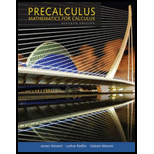Chapter 6.5, Problem 35EPrecalculus: Mathematics for Calcu...

7th Edition
James Stewart + 2 others
ISBN: 9781305071759

Solutions

Chapter
SectionPrecalculus: Mathematics for Calcu...

7th Edition
James Stewart + 2 others
ISBN: 9781305071759
Textbook Problem

The Leaning Tower of Pisa The bell lower of the cathedral in Pisa, Italy, leans 5.6° from the vertical. A tourist stands 105 m from its base, with the tower leaning directly toward her. She measures the angle of elevation to the top of the tower to be 29.2°. Find the length of the tower to the nearest meter.

To determine

To find: The length of the tower.

Explanation

Given:

The angle measure at which the tower leans is 5.6 , distance of tourist from the base of the tower is 105m and the angle of elevation is 29.2° .

Calculation:

The below figure shows triangle ABC .

Figure (1)

From figure (1) find the angle measure of ABC .

The angle measure of ABC is

ABC=DBCDBA=90°5.6°(DBC=90°,DBA=5.6°)=84.4°

The angle measure of ABC is 84.4° .

Use Angle Sum Property in triangle ABC to find the value of angle BAC .

ABC+BCA+BAC=180°BAC=180°(ABC+BCA)(ABC=84

Still sussing out bartleby?

Check out a sample textbook solution.

See a sample solution

The Solution to Your Study Problems

Bartleby provides explanations to thousands of textbook problems written by our experts, many with advanced degrees!

Get Started

Solve the equations in Exercises 126. 6x(x2+1)2(x2+2)48x(x2+1)3(x2+2)3(x2+2)8=0

Finite Mathematics and Applied Calculus (MindTap Course List)

Describe the scores in a sample that has standard deviation of zero.

Statistics for The Behavioral Sciences (MindTap Course List)

In problems 45-62, perform the indicated operations and simplify. 48.

Mathematical Applications for the Management, Life, and Social Sciences

Solve for if 0360. sin3cos=3

Trigonometry (MindTap Course List)